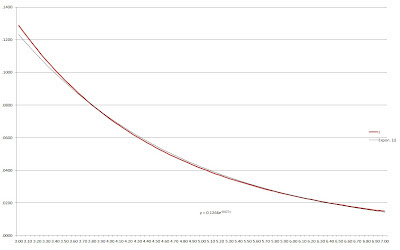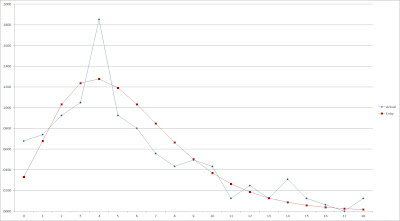## Monday, July 16, 2012

### On Run Distributions, pt. 6: Series Review

This post won’t introduce anything new--instead I’m just going to summarize what I’ve already done, giving you a full example of how to calculate the Enby distribution estimates for a given R/G level. I’ll also provide a spreadsheet with the parameters for each .05 R/G increment between 3-7 so that you don’t have to do all these calculations yourself.

Let’s suppose we have a team that averages exactly 5 R/G (in fact, there is such a team in my sample data--the 1984 Red Sox), and we’d like to estimate their game-level scoring distribution using the Enby distribution methodology. The first step is to estimate the variance of their runs scored per game:

Step 1: Estimate the variance of runs scored per game.

Variance = 1.43*(R/G) + .1345*(R/G)^2 = 1.43*5 + .1345*5^2 = 10.5125

Step 2: Use the mean and variance to estimate the parameters (r and B) of the negative binomial distribution (these formulas are equivalent to what I’ve presented before as explained below):

B = .1345*(R/G) + .43 = .1345*5 + .43 = 1.1025
r = (R/G)/(.1345*(R/G) + .43) = 5/(.1345*5 + .43) = 4.5351

Step 3: Use the negative binomial distribution to estimate the probability of scoring 0 runs:

q(0) = (1 + B)^(-r) = (1 + 1.1025)^(-4.5351) = .0344 (call this value a for ease later on)

Step 4: Use the Tango Distribution to estimate the probability of being shutout, which is equal to the Enby distribution (zero-modified negative binomial) parameter z:

RI = (R/G)/9 = 5/9 = .5556
z = (RI/(RI + .767*RI^2))^9 = .0410

Step 5: Using your spreadsheet, use trial and error (or a solver if you have that that level of functionality) to estimate a new value of r. In choosing this value, you need to ensure that the average R/G predicted by the Enby distribution equals your sample R/G (5 in this case). This needs to be done simultaneously; use the following formula to estimate the initial probability:

q(k) = (r)(r + 1)(r + 2)(r + 3)...(r + k - 1)*B^k/(k!*(1 + B)^(r + k)) for k >=1

Then modify it as follows:
p(0) = z
p(k) = (1 - z)*q(k)/(1 - a)for k >=1

The mean is calculated:
p(1) + 2*p(2) + 3*p(3) + 4*p(4) + ...

The new value of r is the value that, when used in conjunction with this methodology and the previously calculated values for B and z, produce a mean equal to the desired R/G (5 in this case, with a corresponding r of 4.571.

So we have determined that the Enby distribution for a team that scores 5 R/G has parameters (B = 1.1025, r = 4.571, z = .041). The formulas for p(0) and p(k) calculate the probability of scoring k runs in a game.

How does our plot for the 1984 Red Sox compare to their actual scoring output?Of course, we don’t expect a great fit for every team-season. Even if we assumed that there were no variations in run distribution due to the characteristics of an offense, the 162 game sample size would cause deviation from the expected values.
I have calculated the three parameters at each interval of .05 R/G between 3 and 7. While we have some reason to believe that the Enby may be semi-accurate outside of normal ranges, I’m not going to recommend its usage outside of the scoring range of normal teams. Getting a lot more precise than .05 is probably overkill as well, but given my limitation in having to solve for r by trial and error, I’m also limiting the gradients as a matter of practicality.

Here is a link to the spreadsheet. Enter your R/G (only values between 3 and 7 are supported) in the shaded yellow cell. The spreadsheet will round this to the nearest .05 for you. P(k) is the probability of scoring k runs in the game, r is for computation purposes (it is the product of r*(r + 1)*(r + 2)... as applicable), and nb is the probability from the normal distribution without zero modification.

Since I now have a table with the parameters over the 3-7 R/G range, it would feel inappropriate not to make scatterplots and look for patterns. First, z against R/G:The red line is the z values; the thin black line is an exponential regression line that is a decent match for the data over this range. z is the parameter that needs the least investigation, though, as it is calculated via a formula based on the Tango Distribution. The formula makes sense, and there’s no mystery about why it works. The regression equation is superfluous and will certainly fail at low levels of R/G (it will predict that a team that averages 0 R/G will only be shutout in 12.66% of games).

Here is B against R/G:B is a linear function of R/G. This is also not a surprise. Remember that B = variance/mean - 1, but I’m estimating the variance as a function of the mean. In fact, B can be simplified to B = .1345*(R/G) + .43, keeping in mind that I have used a fairly crude estimator of the variance, which is an area that might well be improved upon.

The parameter for which behavior is not defined by a formula is r:Over this range, r is almost linear as a function of R/G. It can be modeled very closely over this range by a quadratic regression. I wouldn’t want to assume that a function can be used to estimate r consistently over a wider range of R/G, and even if it did, I wouldn’t want to advocate it as the value of r should be chosen to ensure that the expected R/G equals the actual R/G. In any event, it’s interesting to see how the parameters might behave in relation to R/G.

This post is running a little shorter than most of the others, so I’ll throw in something that would have gone in the odds and ends post that will close this series. For the last few years I’ve been looking at runs scored and allowed distributions at the end of each season, and in that time the most interesting team I’ve seen is the 2011 Red Sox. Boston led the majors in runs scored, but based on the empirical W% by runs scored in the majors in 2011, their actual distribution of runs scored would have led to an estimated 6.2 less wins than one would assume from just looking at their average runs scored. I thought it would be interesting to look at such a team again with the Enby distribution.

Boston averaged 5.4 R/G, which from the table above means their run distribution will be estimated as Enby(B = 1.1563, r = 4.7, z = .0331). Graphing their actual distribution against the expected, we get this:The Red Sox were shutout a lot more than we’d expect, and while we expected the mode of their runs per game to be 4, they actually scored 4 runs in 18.5% of their games compared to an expectation of 12.8%. They also were clearly below expectation in games of 5-8 runs scored, which are games that a team has a very good chance of winning. The distribution skewed more to right than expected, games in which gaudy runs scored totals have much less of an impact on wins as the marginal value of each run is quite low.

Another way to visualize this (and as you can tell from this series, charts aren’t really my thing--I'm using a lot here, but not to great effect and only because I think tables of numbers would bore you and require more exposition) is to graph the cumulative percentage of team runs scored as we progressively add in games in which k runs were scored.

Boston was shutout eleven times; obviously those games contributed zero runs. They scored one run twelve times, which contributed 12 runs. They scored 875 runs overall, so this represented 1.37% of their total output. They scored two runs fifteen times, for a total of 30 runs. So games with 0-2 runs represented 42/875 = 4.8% of their total output. Continuing in this vein, we can get a graphical sense of the share of their runs that came on the tails of the distribution:I’ve included the Enby distribution expectation as well as the overall 2011 major league average on the graph. The average major league team tallied 88.6% of its runs in games in which ten or less runs were scored, while we’d expect a team that averages 5.4 R/G to have scored 80% of its runs in such games. However, Boston only scored 71.7% of its runs in those contests.